### Home > A2C > Chapter 12 > Lesson 12.1.3 > Problem12-58

12-58.
1. Write an equation of the ellipse with the given information. Homework Help ✎

1. x-intercepts at (±3, 0) and y-intercepts at (0, ±6).

2. x-intercepts at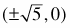and y-intercepts at (0, ±1).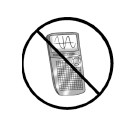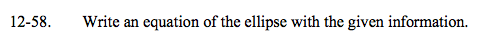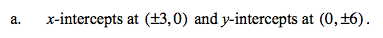$\frac{x^2}{9}+\frac{y^2}{36}=1$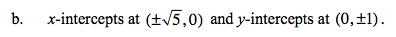See part (a).